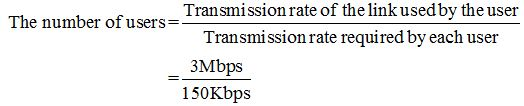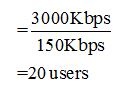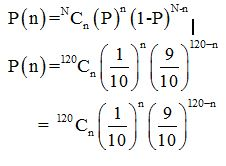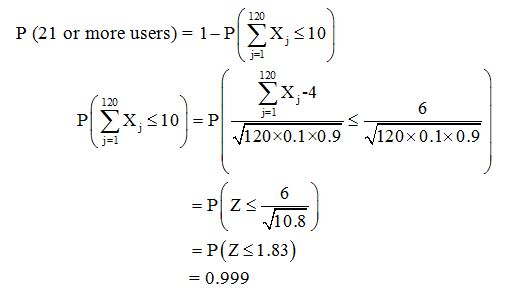SHARE
HELP

To start with, Sr2Jr’s first step is to reduce the expenses related to education. To achieve this goal Sr2Jr organized the textbook’s question and answers. Sr2Jr is community based and need your support to fill the question and answers. The question and answers posted will be available free of cost to all.Authors:
James F. Kurose, Keith W. Ross
Chapter:
Computer Networks And The Internet
Exercise:
Problems
Question:8 | ISBN:9780132856201 | Edition: 6

###Question

Suppose users share a 3 Mbps link. Also suppose each user requires 150 kbps when transmitting, but each user transmits only 10 percent of the time. (See the discussion of packet switching versus circuit switching in Section 1.3.)

a. When circuit switching is used, how many users can be supported?

b. For the remainder of this problem, suppose packet switching is used. Find the probability that a given user is transmitting.

c. Suppose there are 120 users. Find the probability that at any given time, exactly n users are transmitting simultaneously. (Hint: Use the binomial distribution.)

d. Find the probability that there are 21 or more users transmitting simultaneously.

###Answer

a)We know that  1Mbps=10 bpsSo, the total number of users=20.

b)

Transmission rate=10%

probability=1/10=0.1

c)d)So, P (21 or more users) =1-0.999 = 0.001(approx.)

### Discussions

Post the discussion to improve the above solution.